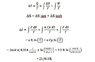# Calculate the entropy changes of the system and the surroundings

• sara lopez

## Homework Statement

Calculate the entropy changes of the system and the surroundings if the initial and final states are the same
as in part a ( part a= 2.000 mol of neon (assume ideal with CV,m _ 3R/2) is expanded isothermally at 298.15K from 2.000 atm pressure to 1.000 atm pressure and is then heated from 298.15K to 398.15K at a constant pressure of 1.000 atm) , but if the gas is expanded irreversibly and isothermally against an external pressure of 1.000 atm and then heated irreversibly with the surroundings remaining essentially at equilibrium at 400 K.

2.000 mol of neon

CV,m = 3R/2

T1= 298.15K

T2= 400 K.

P1= 2.000 atm v1= 24,4 L

P2=Pext = 1.000 atm v2= 48,89L

[/B]

## The Attempt at a Solution

I think I am getting a bit confused here because the problem asks for the entropy changes of the system and the surroundings, but how to calculate the surroundings for irreversibly?

ΔS u = ΔS sist + ΔS surro

ΔSsurroundings = qsurroundings /Tsurroundings = − q system /T surrounding

#### Attachments

•eq1.jpg
7.5 KB · Views: 438